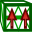# Adding a Solution Coefficient Source

Apply a solution coefficient data definition and use as an impressed current source at a specified point in space.

1. On the Source/Load tab, in the Equivalent Sources group, click theSolution Coefficient icon.
2. In the Magnitude scale factor field, specify the scaling factor.
3. In the Phase offset (degrees) field, specify the phase (in degrees) to be added to the phase of the currents.
4. In the Solution coefficient data field, specify the solution coefficient data to be used to define the solution coefficient source.
5. In the Position field, specify where the source is to be placed.
6. In the Label field, add a unique label for the solution coefficient data source.
7. Click the Create button to create the solution coefficient source and to close the dialog.